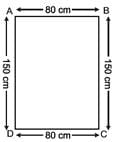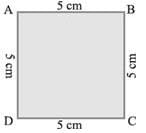Important Questions Test: Mensuration

# Important Questions Test: Mensuration

Test Description

## 20 Questions MCQ Test NCERT Mathematics for CAT Preparation | Important Questions Test: Mensuration

Important Questions Test: Mensuration for Class 6 2022 is part of NCERT Mathematics for CAT Preparation preparation. The Important Questions Test: Mensuration questions and answers have been prepared according to the Class 6 exam syllabus.The Important Questions Test: Mensuration MCQs are made for Class 6 2022 Exam. Find important definitions, questions, notes, meanings, examples, exercises, MCQs and online tests for Important Questions Test: Mensuration below.
Solutions of Important Questions Test: Mensuration questions in English are available as part of our NCERT Mathematics for CAT Preparation for Class 6 & Important Questions Test: Mensuration solutions in Hindi for NCERT Mathematics for CAT Preparation course. Download more important topics, notes, lectures and mock test series for Class 6 Exam by signing up for free. Attempt Important Questions Test: Mensuration | 20 questions in 20 minutes | Mock test for Class 6 preparation | Free important questions MCQ to study NCERT Mathematics for CAT Preparation for Class 6 Exam | Download free PDF with solutions
 1 Crore+ students have signed up on EduRev. Have you?
Important Questions Test: Mensuration - Question 1

### The perimeter of a square is 100 cm. Its side is

Important Questions Test: Mensuration - Question 2

### If the perimeter of a square is 44 cm, then its area is

Important Questions Test: Mensuration - Question 3

### If the perimeter of a square is 16 cm, then its area is

Important Questions Test: Mensuration - Question 4

The side of a square is 8 cm. If its side is doubled, then its new perimeter is

Important Questions Test: Mensuration - Question 5

The length and breadth of a rectangle are 10 cm and 8 cm respectively. If its length is doubled, then its new area is

Important Questions Test: Mensuration - Question 6

Area of a rectangle = ________

Important Questions Test: Mensuration - Question 7

Find the area of a square plot of side 7 m.

Detailed Solution for Important Questions Test: Mensuration - Question 7

Side of Square = a2

(7)2

= 49 m2

Important Questions Test: Mensuration - Question 8

__________ is the distance covered along the boundary forming a closed figure when you go round the figure once.

Important Questions Test: Mensuration - Question 9

Perimeter of a square = ________ × length of a side

Important Questions Test: Mensuration - Question 10

Meera went to a park 150 m long and 80 m wide. She took one complete round on its boundary. What is the distance covered by her?

Detailed Solution for Important Questions Test: Mensuration - Question 10Let ABCD is a park whose lengths are BC, AD and widths are AB, CD, respectively. Hence, AB = CD = 80 m. and BC = DA = 150m Now, the sum of the lengths of four sides

=AB + BC + CD + DA

= 80 m + 150 m + 80 m + 150 m

= (80 + 150 + 80 + 150) m

=460 m

Perimeter of the park = sum of the lengths of four sides of the park = 460 m

Hence, distance covered by Meera is 460 m.

Important Questions Test: Mensuration - Question 11

A room is 4 m long and 3 m 50 cm wide. How many square metres of carpet is needed to cover the floor of the room?

Important Questions Test: Mensuration - Question 12

Find the area of square whose perimeter is 48cm

Important Questions Test: Mensuration - Question 13

Find the perimeter of a regular pentagon with each side measuring 3 cm.

Important Questions Test: Mensuration - Question 14

What is the perimeter of the following figure ?Important Questions Test: Mensuration - Question 15

Find the perimeter of an isosceles triangle with equal sides 8 cm each and third side 6 cm.

Important Questions Test: Mensuration - Question 16

Perimeter of a rectangle = _________ × (length + breadth)

Important Questions Test: Mensuration - Question 17

Perimeter of an __________ = 3 × length of a side

Important Questions Test: Mensuration - Question 18

A farmer has a rectangular field of length and breadth 240 m and 180 m respectively. He wants to fence it with 3 rounds of rope. What is the total length of rope he must use?

Important Questions Test: Mensuration - Question 19

If the area of rectangle increases from 2 cm2 to 4 cm2 the perimeter will

Important Questions Test: Mensuration - Question 20

Which figure encloses more area: a square of side 2 cm ; a rectangle of side 3 cm and 2 cm ;An equilateral triangle of side 4 cm?

## NCERT Mathematics for CAT Preparation

276 docs|149 tests
 Use Code STAYHOME200 and get INR 200 additional OFF Use Coupon Code
Information about Important Questions Test: Mensuration Page
In this test you can find the Exam questions for Important Questions Test: Mensuration solved & explained in the simplest way possible. Besides giving Questions and answers for Important Questions Test: Mensuration, EduRev gives you an ample number of Online tests for practice

## NCERT Mathematics for CAT Preparation

276 docs|149 tests